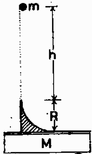## Our Pick(s) Job begins : 08 Oct 20, See the solutions : 09 Oct 20

One step back

1. A ℓ long rope protrudes from a U-shaped, narrow, small, fixed tube facing downwards, as shown. Initially, the rope is ℓ / 2 long on both sides. Here it is in an unstable equilibrium position as the friction is negligible.a)    If the rope starts to slide to one side, what will be its speed by which time will it straighten?

b) What is the speed of the rope when the instantaneous center of mass of the rope is just at the same height as the end of the upward sliding piece of rope?

Start Thinking

2. At what angle do we have to throw a ball from the bottom of a slope with a slope of 300 if we want it to rise vertically after an elastic collision with the slope?

Similar Shot

3. A slope with a quarter-circular cross-section and a radius R = 0.5 m, tangentially connected to a plank, weighing M = 6 kg(together with the structure of circular cross section), as shown in the figure. From a height h = 1.5 m from the top of the slope, a body of negligible size weighing m = 3 kg falls on the slope, through which it slides without friction.

a. At what maximum force does the  if the plank can move freely without friction?

b. What is the radius of curvature of the trajectory of the ball relative to the plank at the bottom of the arc?Relax
4. A soccer ball approaches the player at a speed of 8 m / s, who "wants to handle" the ball. How fast does the player's foot have to move in order for the ball to stop after the collision? Consider the impact to be perfectly elastic, and note that the weight of the foot is much greater than the weight of the ball.
Mission Accomplished
5. A body of mass M with a half-cylindrical dent on one side stands on a frictionless table, as shown in the figure. What is the minimum initial speed v required for the body of mass m so that it runs through the dent of radius R of the body of mass M? (Friction is negligible everywhere.)